NCERT Exemplar - MCQs

Chapter 6 Class 12 Application of Derivatives
Serial order wise

## (C) cos x                            (D) cos 3x

This question is similar to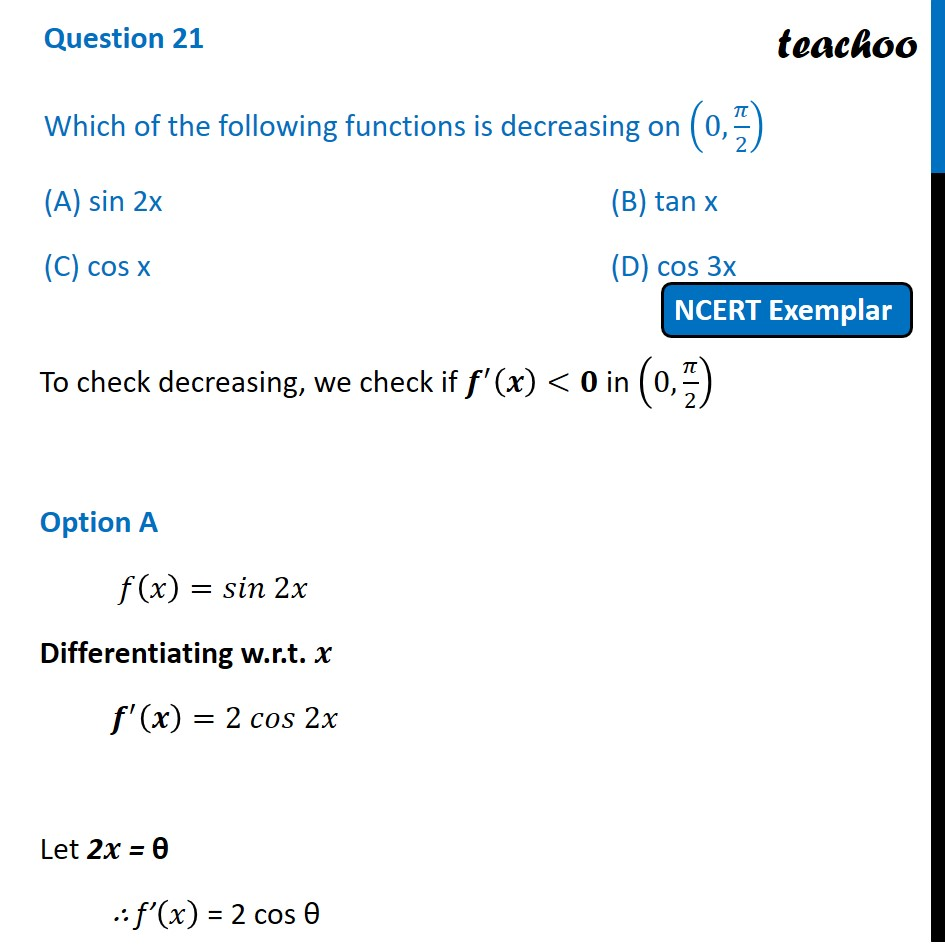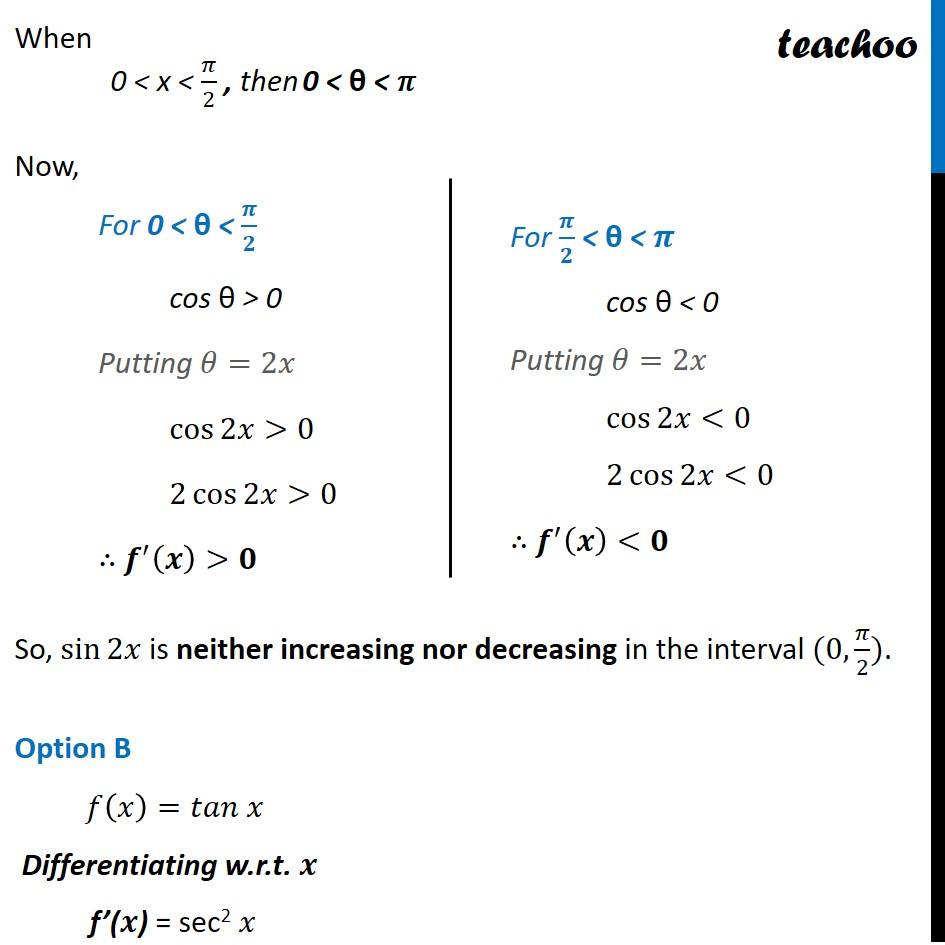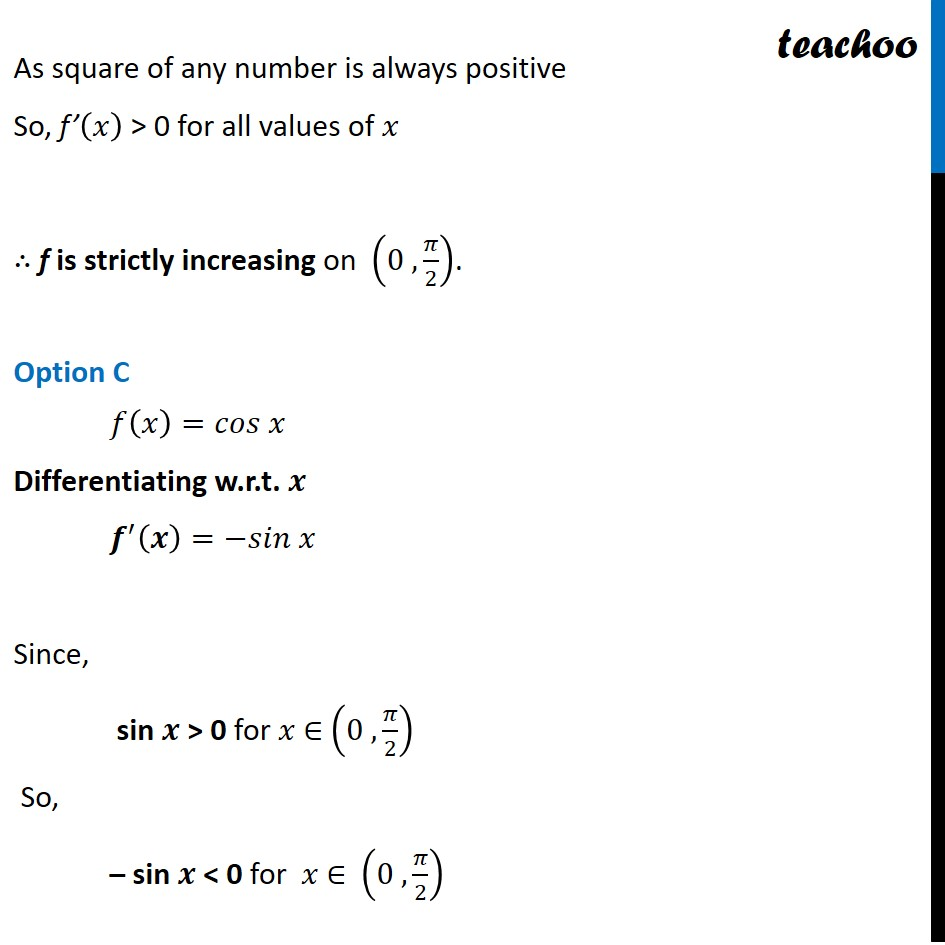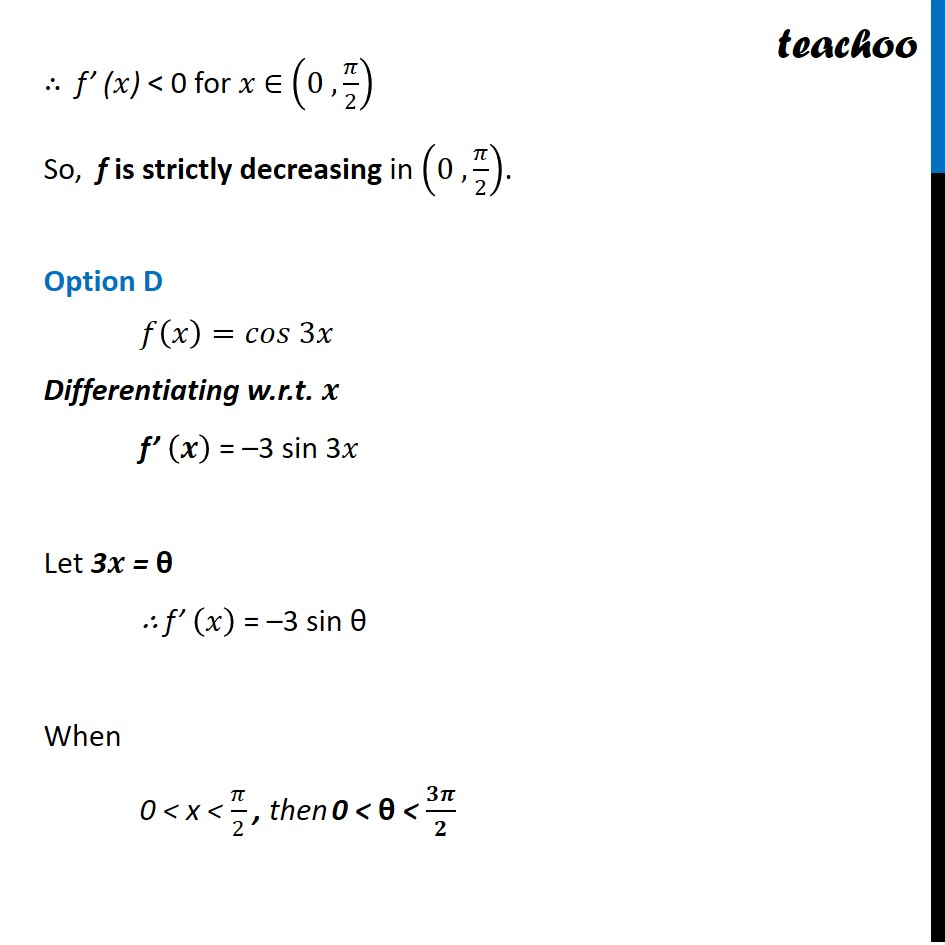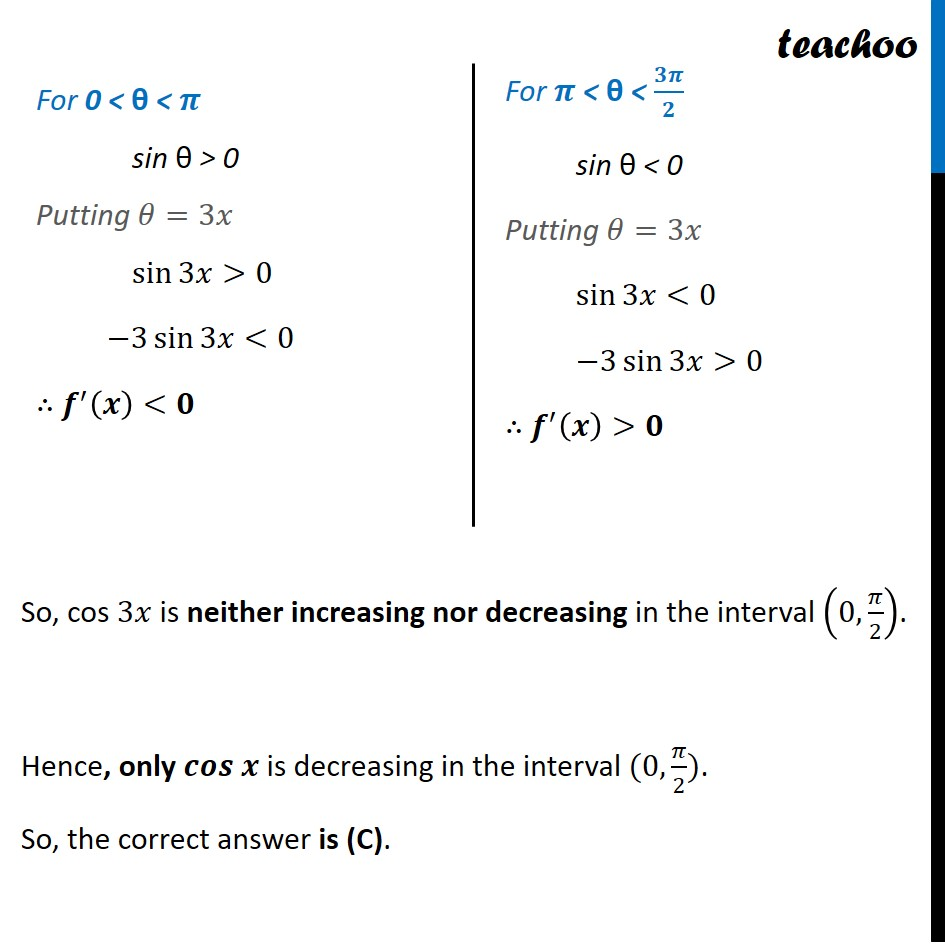Get live Maths 1-on-1 Classs - Class 6 to 12

### Transcript

Question 21 Which of the following functions is decreasing on (0,𝜋/2) (A) sin 2x (B) tan x (C) cos x (D) cos 3x To check decreasing, we check if 𝒇^′ (𝒙)<𝟎 in (0,𝜋/2) Option A 𝑓(𝑥)=𝑠𝑖𝑛 2𝑥 Differentiating w.r.t. 𝒙 𝒇^′ (𝒙)=2 𝑐𝑜𝑠 2𝑥 Let 2𝒙 = θ ∴ f’(𝑥) = 2 cos θ When 0 < x < 𝜋/2 , then 0 < θ < 𝜋 Now, So, sin⁡2𝑥 is neither increasing nor decreasing in the interval (0,𝜋/2). Option B 𝑓(𝑥)=𝑡𝑎𝑛 𝑥 Differentiating w.r.t. 𝒙 f’(𝒙) = sec2 𝑥 For 0 < θ < 𝝅/𝟐 cos θ > 0 Putting 𝜃=2𝑥 cos⁡2𝑥>0 2 cos⁡2𝑥>0 ∴ 𝒇^′ (𝒙)>𝟎 For 𝝅/𝟐 < θ < 𝝅 cos θ < 0 Putting 𝜃=2𝑥 cos⁡2𝑥<0 2 cos⁡2𝑥<0 ∴ 𝒇^′ (𝒙)<𝟎 As square of any number is always positive So, f’(𝑥) > 0 for all values of 𝑥 ∴ f is strictly increasing on (0 , 𝜋/2). Option C 𝑓(𝑥)=𝑐𝑜𝑠 𝑥 Differentiating w.r.t. 𝒙 𝒇^′ (𝒙)=−𝑠𝑖𝑛 𝑥 Since, sin 𝒙 > 0 for 𝑥 ∈ (0 , 𝜋/2) So, – sin 𝒙 < 0 for 𝑥 ∈ (0 , 𝜋/2) ∴ f’ (𝑥) < 0 for 𝑥 ∈ (0 , 𝜋/2) So, f is strictly decreasing in (0 , 𝜋/2). Option D 𝑓(𝑥)=𝑐𝑜𝑠 3𝑥 Differentiating w.r.t. 𝒙 f’ (𝒙) = –3 sin 3𝑥 Let 3𝒙 = θ ∴ f’ (𝑥) = –3 sin θ When 0 < x < 𝜋/2 , then 0 < θ < 𝟑𝝅/𝟐 For 0 < θ < 𝝅 sin θ > 0 Putting 𝜃=3𝑥 sin⁡3𝑥>0 −3 sin⁡3𝑥<0 ∴ 𝒇^′ (𝒙)<𝟎 For 𝝅 < θ < 𝟑𝝅/𝟐 sin θ < 0 Putting 𝜃=3𝑥 sin⁡3𝑥<0 −3 sin⁡3𝑥>0 ∴ 𝒇^′ (𝒙)>𝟎 So, cos 3𝑥 is neither increasing nor decreasing in the interval (0,𝜋/2). Hence, only 𝒄𝒐𝒔 𝒙 is decreasing in the interval (0,𝜋/2). So, the correct answer is (C).